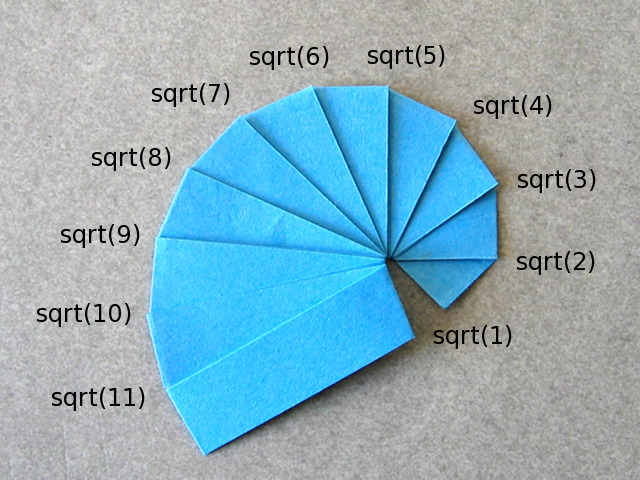# Pythagorean Spiral

Folder:
Model type:
Spirals
GeometryPaper: 1cm by 50cm
Model: width of 5.2cm, height of 3.7cm

This is a fold that constructs consecutive square roots. It's actually a nice exercise for kids learning about the Pythagorean theorem (a^2 + b^2 = c^2 in a right-angled triangle).

### a great help

good model and gives a great help to every one specially for children. to give them better understanding